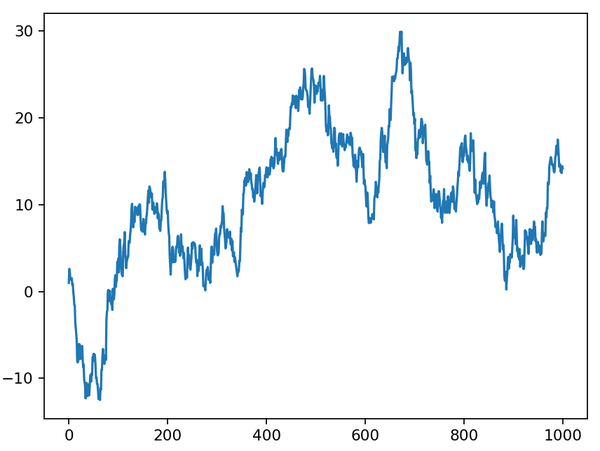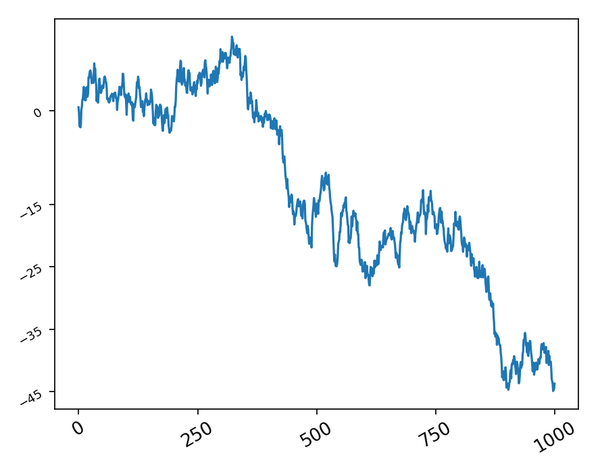# 坐标轴刻度

```plt.plot(np.random.randn(1000).cumsum())
``````plt.plot(np.random.randn(1000).cumsum())
plt.xticks([0,250,500,750,1000],rotation=30, fontsize='large')
plt.yticks([-45,-35,-25,-15,0],rotation=30, fontsize='small')
```